Courses

# Olympiad Test: Patterns - 1

## 15 Questions MCQ Test Science Olympiad for Class 5 | Olympiad Test: Patterns - 1

Description
This mock test of Olympiad Test: Patterns - 1 for Class 5 helps you for every Class 5 entrance exam. This contains 15 Multiple Choice Questions for Class 5 Olympiad Test: Patterns - 1 (mcq) to study with solutions a complete question bank. The solved questions answers in this Olympiad Test: Patterns - 1 quiz give you a good mix of easy questions and tough questions. Class 5 students definitely take this Olympiad Test: Patterns - 1 exercise for a better result in the exam. You can find other Olympiad Test: Patterns - 1 extra questions, long questions & short questions for Class 5 on EduRev as well by searching above.
QUESTION: 1

### In this question, various terms are missing as shown by(?). Choose the missing term out of the given alternatives.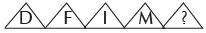Solution: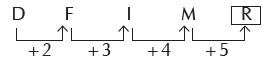So, the next letter in the series will
be R.

QUESTION: 2

### In this question, various terms are missing as shown by(?). Choose the missing term out of the given alternatives. PZA QYC RXE SWG ?

Solution:

It is a combination of 3 series i.e.
P, Q, R, S, ?
Z, Y,X, W, ?
A, C, E G, ?

so,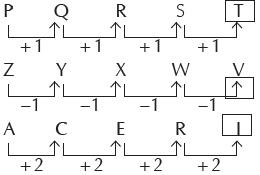So, the next term will be TVI

QUESTION: 3

### In this question, various terms are missing as shown by(?). Choose the missing term out of the given alternatives. K5M   I8P   G11S   E14V   ?

Solution:

It is a combination of 3 series.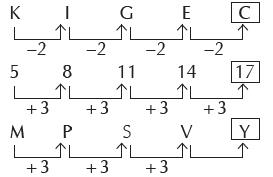QUESTION: 4

In this question, various terms are missing as shown by(?). Choose the missing term out of the given alternatives.

AEI   BFJ   CGK   ?

Solution:

It is a combination of 3 series.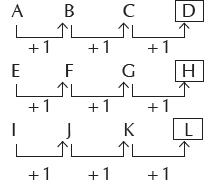So, next term will be DHL

QUESTION: 5

In this question, various terms are missing as shown by(?). Choose the missing term out of the given alternatives.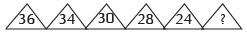Solution: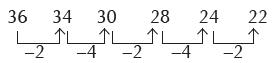QUESTION: 6

In this question, various terms are missing as shown by(?). Choose the missing term out of the given alternatives.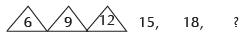Solution: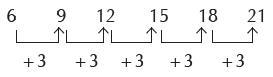QUESTION: 7

In this question, various terms are missing as shown by(?). Choose the missing term out of the given alternatives.

9, 17, 29, 45, ?

Solution: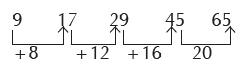QUESTION: 8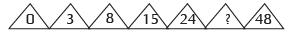Solution:

12 – 1 = 0
22 – 1 = 3
32 – 1 = 8
42 – 1 = 15
52 –1 = 24
62 – 1= 35
72 –1 = 48

QUESTION: 9

EJO   FKP   GLQ   HMR   ?

Solution: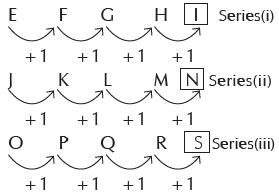QUESTION: 10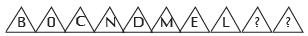Solution: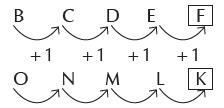QUESTION: 11

Find the pattern among the answer figures which completes the problem figure (X)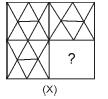Solution:

The pattern which is available in other three squares will complete the problem figure (X)

QUESTION: 12

Garima created the pattern shown here using tooth picks.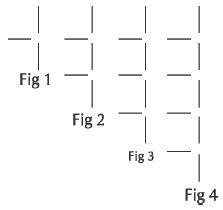If this pattern continues, how many toothpicks are there in figure 8 in the pattern.

Solution:

In the first figure, number of tooth picks =3 and in the second, third and fourth figure, these are 5, 7 and 9 respectively.
So, the pattern followed is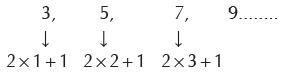So, in the fig. 8, number of tooth
picks = 2×8+1 = 17

QUESTION: 13

Complete the given series: 1, 0, 3, 2, 5, 4, (?)

Solution:

The given series is a combination of
two series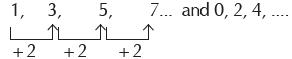QUESTION: 14

Housing board built 100 flats. A painteris engaged to serially number each flatindividually from 1 to 100. How manytimes will he be required to write zero.

Solution:

Clearly, the digit with zero are as
follows-
10, 20, 30, 40, 50, 60, 70, 80,
90,100
So, the number of zeroes are 11.

QUESTION: 15

Which of the following options will complete the fig(X) ?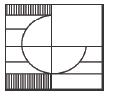Solution:

On seeing carefully, we get that fig.
C completes the fig(X)